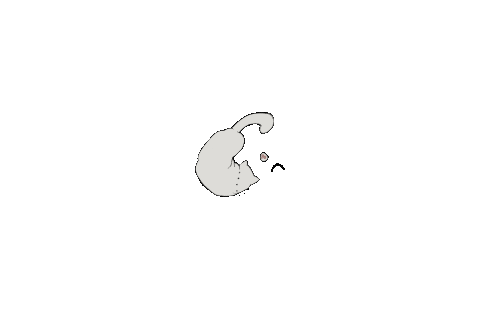# Introduction

In order to measure the effectiveness of our drug and help improve the efficiency of drug production, we can predict the maximum drug loading amount, and in order to reach this goal, we can use the Fick's Laws to help solve this problem. It is known that the drugs are transferred into the liposome through diffusion, and we can assume that:

# Assumption

The drugs have a distance to travel when they are loaded, and the distance would be changed due to the oscillation of the liposome and the drug. In order to make solving the problem easier, we can assume that the distance is the average distance of all the drugs' distance between the liposome when the loading of drugs occur.

# Derivation

we can draw a conclusion of the diffusion constant of doxorubicin and liposome via the Fick's First Law, which is:

$$J=-D▽C$$

From the Fick's First Law, we can know that:

$$D=▽C-J$$

After discovering the diffusion coefficient, we can then work out how many doxorubicins would diffuse into the liposome as time passes, and finally work out the C(concentration) of the doxorubicin in the liposome by the Fick's Second Law, which is:

$$\frac{∂C}{∂t}=D(\frac{∂^2C}{∂r^2}+\frac{2}{r}\frac{∂C}{∂r})$$

After working out the concentration of the doxorubicin, we can find out the A(amount) of it by:

$$A=VC$$

# Conclusion

By identifying the amount of the doxorubicin in the liposome, it can be easier for us to determine how much doxorubicin need to be added to reach the goal of adding enough doxorubicin while not doing much harm to the normal human body cells, which can better help predict the effectiveness of our drug. Moreover, we can further calculate the time which can help us know how long to wait when loading drugs, which can further help us improve the efficiency of drug loading and may be helpful in future mass production of the drug.